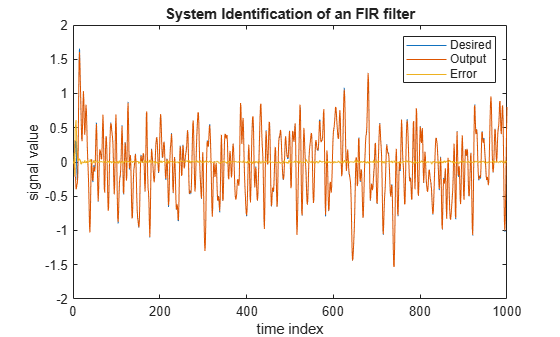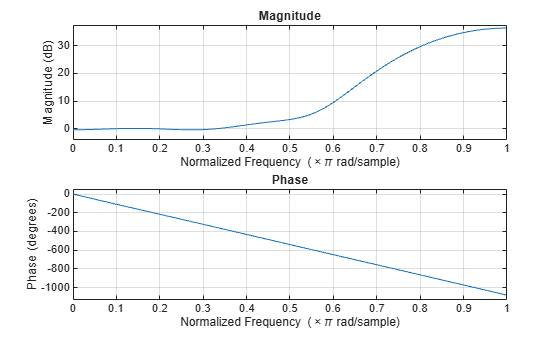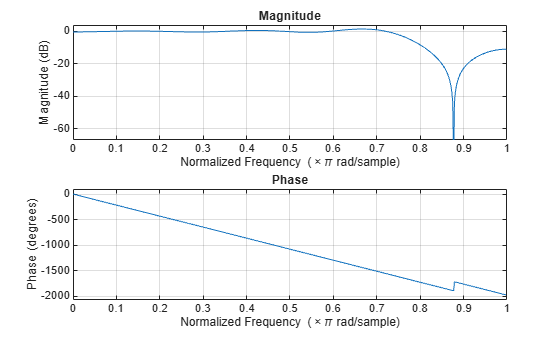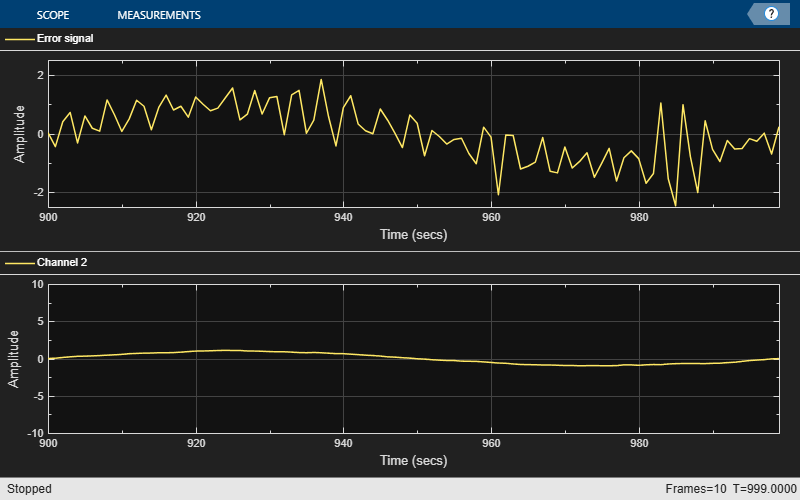# dsp.RLSFilter

Compute output, error and coefficients using recursive least squares (RLS) algorithm

## Description

The `dsp.RLSFilter` System object™ filters each channel of the input using RLS filter implementations.

To filter each channel of the input:

1. Create the `dsp.RLSFilter` object and set its properties.

2. Call the object with arguments, as if it were a function.

## Creation

### Syntax

``rlsFilt = dsp.RLSFilter``
``rlsFilt = dsp.RLSFilter(len)``
``rlsFilt = dsp.RLSFilter(Name,Value)``

### Description

````rlsFilt = dsp.RLSFilter` returns an adaptive RLS filter System object, `rlsFilt`. This System object computes the filtered output, filter error, and the filter weights for a given input and desired signal using the RLS algorithm. ```

example

````rlsFilt = dsp.RLSFilter(len)` returns an RLS filter System object, `rlsFilt`. This System object has the `Length` property set to `len`.```
````rlsFilt = dsp.RLSFilter(Name,Value)` returns an RLS filter System object with each specified property set to the specified value. Enclose each property name in single quotes. Unspecified properties have default values.```

## Properties

expand all

Unless otherwise indicated, properties are nontunable, which means you cannot change their values after calling the object. Objects lock when you call them, and the `release` function unlocks them.

If a property is tunable, you can change its value at any time.

You can specify the method used to calculate filter coefficients as `Conventional RLS`  , `Householder RLS`  , `Sliding-window RLS` , `Householder sliding-window RLS` , or `QR decomposition`  . This property is nontunable.

Specify the length of the RLS filter coefficients vector as a scalar positive integer value. This property is nontunable.

Data Types: `single` | `double` | `int8` | `int16` | `int32` | `int64` | `uint8` | `uint16` | `uint32` | `uint64`

Specify the width of the sliding window as a scalar positive integer value greater than or equal to the `Length` property value. This property is nontunable.

#### Dependencies

This property applies only when the `Method` property is set to `Sliding-window RLS` or ```Householder sliding-window RLS```.

Data Types: `single` | `double` | `int8` | `int16` | `int32` | `int64` | `uint8` | `uint16` | `uint32` | `uint64`

Specify the RLS forgetting factor as a scalar positive numeric value less than or equal to 1. Setting this property value to 1 denotes infinite memory, while adapting to find the new filter.

Tunable: Yes

Data Types: `single` | `double`

Specify the initial values of the FIR adaptive filter coefficients as a scalar or a vector of length equal to the `Length` property value.

Tunable: Yes

Data Types: `single` | `double`

Specify the initial values of the inverse covariance matrix of the input signal. This property must be either a scalar or a square matrix, with each dimension equal to the `Length` property value. If you set a scalar value, the `InverseCovariance` property is initialized to a diagonal matrix with diagonal elements equal to that scalar value.

Tunable: Yes

#### Dependencies

This property applies only when the `Method` property is set to `Conventional RLS` or `Sliding-window RLS`.

Data Types: `single` | `double`

Specify the initial values of the square root inverse covariance matrix of the input signal. This property must be either a scalar or a square matrix with each dimension equal to the `Length` property value. If you set a scalar value, the `SquareRootInverseCovariance` property is initialized to a diagonal matrix with diagonal elements equal to that scalar value.

Tunable: Yes

#### Dependencies

This property applies only when the `Method` property is set to `Householder RLS` or ```Householder sliding-window RLS```.

Data Types: `single` | `double`

Specify the initial values of the square root covariance matrix of the input signal. This property must be either a scalar or a square matrix with each dimension equal to the `Length` property value. If you set a scalar value, the `SquareRootCovariance` property is initialized to a diagonal matrix with diagonal elements equal to the scalar value.

Tunable: Yes

#### Dependencies

This property applies only when the `Method` property is set to `QR-decomposition RLS`.

Data Types: `single` | `double`

Specify whether the filter coefficient values should be locked. When you set this property to `true`, the filter coefficients are not updated and their values remain the same. The default value is `false` (filter coefficients continuously updated).

Tunable: Yes

## Usage

### Syntax

``y = rlsFilt(x,d)``
``````[y,e] = rlsFilt(x,d)``````

### Description

example

````y = rlsFilt(x,d)` recursively adapts the reference input, `x`, to match the desired signal, `d`, using the System object, `rlsFilt`. The desired signal, `d`, is the signal desired plus some undesired noise.```
``````[y,e] = rlsFilt(x,d)``` shows the output of the RLS filter along with the error, `e`, between the reference input and the desired signal. The filters adapts its coefficients until the error `e` is minimized. You can access these coefficients by accessing the `Coefficients` property of the object. This can be done only after calling the object. For example, to access the optimized coefficients of the `rlsFilt` filter, call `rlsFilt.Coefficients` after you pass the input and desired signal to the object.```

### Input Arguments

expand all

The signal to be filtered by the RLS filter. The input, `x`, and the desired signal, `d`, must have the same size and data type.

The input can be a variable-size signal. You can change the number of elements in the column vector even when the object is locked. The System object locks when you call the object to run its algorithm.

Data Types: `single` | `double`
Complex Number Support: Yes

The RLS filter adapts its coefficients to minimize the error, `e`, and converge the input signal `x` to the desired signal `d` as closely as possible.

The input, `x`, and the desired signal, `d`, must have the same size and data type.

The desired signal, `d`, can be a variable-size signal. You can change the number of elements in the column vector even when the object is locked. The System object locks when you call the object to run its algorithm.

Data Types: `single` | `double`
Complex Number Support: Yes

### Output Arguments

expand all

Filtered output, returned as a scalar or a column vector. The object adapts its filter coefficients to converge the input signal `x` to match the desired signal `d`. The filter outputs the converged signal.

Data Types: `single` | `double`
Complex Number Support: Yes

Difference between the output signal `y` and the desired signal `d`, returned as a scalar or a column vector. The objective of the RLS filter is to minimize this error. The object adapts its coefficients to converge toward optimal filter coefficients that produce an output signal that matches closely with the desired signal. For more details on how `e` is computed, see Algorithms. To access the RLS filter coefficients, call `rlsFilt.Coefficients` after you pass the input and desired signal to the object.

Data Types: `single` | `double`
Complex Number Support: Yes

## Object Functions

To use an object function, specify the System object as the first input argument. For example, to release system resources of a System object named `obj`, use this syntax:

`release(obj)`

expand all

 `msesim` Estimated mean squared error for adaptive filters
 `step` Run System object algorithm `release` Release resources and allow changes to System object property values and input characteristics `reset` Reset internal states of System object

## Examples

collapse all

Use a recursive least squares (RLS) filter to identify an unknown system modeled with a lowpass FIR filter. Compare the frequency responses of the unknown and estimated systems.

Note: If you are using R2016a or an earlier release, replace each call to the object with the equivalent `step` syntax. For example, `obj(x)` becomes `step(obj,x)`.

Initialization

Create a `dsp.FIRFilter` object that represents the system to be identified. Pass the signal `x` to the FIR filter. The output of the unknown system is the desired signal `d`, which is the sum of the output of the unknown system (FIR filter) and an additive noise signal `n`.

```filt = dsp.FIRFilter('Numerator',fir1(10,.25)); x = randn(1000,1); n = 0.01*randn(1000,1); d = filt(x) + n;```

Create a `dsp.RLSFilter` object to create an RLS filter. Set the length of the filter to 11 taps and the forgetting factor to 0.98. Pass the primary input signal `x` and the desired signal `d` to the RLS filter. The output `y` of the adaptive filter is the signal converged to the desired signal `d` thereby minimizing the error `e` between the two signals.

```rls = dsp.RLSFilter(11, 'ForgettingFactor', 0.98); [y,e] = rls(x,d); w = rls.Coefficients;```

Plot the results

The output signal matches the desired signal, making the error between the two close to zero.

```plot(1:1000, [d,y,e]); title('System Identification of an FIR filter'); legend('Desired', 'Output', 'Error'); xlabel('time index'); ylabel('signal value');```Compare the weights

The weights vector `w` represents the coefficients of the RLS filter that is adapted to resemble the unknown system (FIR filter). To confirm the convergence, compare the numerator of the FIR filter and the estimated weights of the RLS filter.

The estimated filter weights closely match the actual filter weights, confirming the results seen in the previous signal plot.

```stem([filt.Numerator; w].'); legend('Actual','Estimated'); xlabel('coefficient #'); ylabel('coefficient value');```This example demonstrates the RLS adaptive algorithm using the inverse system identification model shown here.Cascading the adaptive filter with an unknown filter causes the adaptive filter to converge to a solution that is the inverse of the unknown system.

If the transfer function of the unknown system and the adaptive filter are H(z) and G(z), respectively, the error measured between the desired signal and the signal from the cascaded system reaches its minimum when G(z)$×$H(z) = 1. For this relation to be true, G(z) must equal 1/H(z), the inverse of the transfer function of the unknown system.

To demonstrate that this is true, create a signal s to input to the cascaded filter pair.

`s = randn(3000,1);`

In the cascaded filters case, the unknown filter results in a delay in the signal arriving at the summation point after both filters. To prevent the adaptive filter from trying to adapt to a signal it has not yet seen (equivalent to predicting the future), delay the desired signal by 12 samples, which is the order of the unknown system.

Generally, you do not know the order of the system you are trying to identify. In that case, delay the desired signal by number of samples equal to half the order of the adaptive filter. Delaying the input requires prepending 12 zero-value samples to the input `s`.

```delay = zeros(12,1); d = [delay; s(1:2988)]; % Concatenate the delay and the signal.```

You have to keep the desired signal vector `d` the same length as `x`, so adjust the signal element count to allow for the delay samples.

Although not generally the case, for this example you know the order of the unknown filter, so add a delay equal to the order of the unknown filter.

For the unknown system, use a lowpass, 12th-order FIR filter.

```filt = dsp.FIRFilter; filt.Numerator = fir1(12,0.55,'low');```

Filtering `s` provides the input data signal for the adaptive algorithm function.

`x = filt(s);`

To use the RLS algorithm, create a `dsp.RLSFilter` object and set its `Length`, `ForgettingFactor`, and `InitialInverseCovariance` properties.

For more information about the input conditions to prepare the RLS algorithm object, refer to `dsp.RLSFilter`.

```p0 = 2 * eye(13); lambda = 0.99; rls = dsp.RLSFilter(13,'ForgettingFactor',lambda,... 'InitialInverseCovariance',p0);```

This example seeks to develop an inverse solution, you need to be careful about which signal carries the data and which is the desired signal.

Earlier examples of adaptive filters use the filtered noise as the desired signal. In this case, the filtered noise (`x`) carries the unknown system's information. With Gaussian distribution and variance of 1, the unfiltered noise `d` is the desired signal. The code to run this adaptive filter is:

`[y,e] = rls(x,d);`

where `y` returns the filtered output and `e` contains the error signal as the filter adapts to find the inverse of the unknown system.

Obtain the estimated coefficients of the RLS filter.

`b = rls.Coefficients;`

View the frequency response of the adapted RLS filter (inverse system, G(z)) using `freqz`. The inverse system looks like a highpass filter with linear phase.

`freqz(b,1)`View the frequency response of the unknown system, H(z). The response is that of a lowpass filter with a cutoff frequency of 0.55.

`freqz(filt.Numerator,1)`The result of the cascade of the unknown system and the adapted filter is a compensated system with an extended cutoff frequency of 0.8.

```overallCoeffs = conv(filt.Numerator,b); freqz(overallCoeffs,1)```Cancel additive noise n added to an unknown system using an RLS filter. The RLS filter adapts its coefficients until its transfer function matches the transfer function of the unknown system as closely as possible. The difference between the output of the adaptive filter and the output of the unknown system is the error signal `e`, which represents the additive white noise. Minimizing this error signal is the objective of the adaptive filter.

Note: If you are using R2016a or an earlier release, replace each call to the object with the equivalent step syntax. For example, `obj(x)` becomes `step(obj,x)`.

Initialization

Create a `dsp.FIRFilter` System object™ to represent the unknown system. Create a `dsp.RLSFilter` object and set the length to 11 taps. Set the method to `'Householder RLS'`. Create a sine wave to represent the noise added to the unknown system. View the signals in a time scope.

```FrameSize = 100; NIter = 10; rls = dsp.RLSFilter('Length',11,... 'Method','Householder RLS'); filt = dsp.FIRFilter('Numerator',... fir1(10,[.5,.75])); sinewave = dsp.SineWave('Frequency',0.01,... 'SampleRate',1,... 'SamplesPerFrame',FrameSize); scope = timescope('LayoutDimensions',[2 1],... 'NumInputPorts',2, ... 'TimeUnits','Seconds',... 'YLimits',[-2.5 2.5], ... 'BufferLength',2*FrameSize*NIter,... 'ActiveDisplay',1,... 'ShowLegend',true,... 'ChannelNames',{'Noisy signal'},... 'ActiveDisplay',2,... 'ShowLegend',true,... 'ChannelNames',{'Error signal'}); for k = 1:NIter x = randn(FrameSize,1); d = filt(x) + sinewave(); [y,e] = rls(x,d); w = rls.Coefficients; scope(d,e) end release(scope)```## Algorithms

The `dsp.RLSFilter` System object, when `Conventional RLS` is selected, recursively computes the least squares estimate (RLS) of the FIR filter weights. The System object estimates the filter weights or coefficients, needed to convert the input signal into the desired signal. The input signal can be a scalar or a column vector. The desired signal must have the same data type, complexity, and dimensions as the input signal. The corresponding RLS filter is expressed in matrix form as P(n) :

`$\begin{array}{l}k\left(n\right)=\frac{{\lambda }^{-1}P\left(n-1\right)u\left(n\right)}{1+{\lambda }^{-1}{u}^{H}\left(n\right)P\left(n-1\right)u\left(n\right)}\\ y\left(n\right)={w}^{T}\left(n-1\right)u\left(n\right)\\ e\left(n\right)=d\left(n\right)-y\left(n\right)\\ w\left(n\right)=w\left(n-1\right)+{k}^{*}\left(n\right)e\left(n\right)\\ P\left(n\right)={\lambda }^{-1}P\left(n-1\right)-{\lambda }^{-1}k\left(n\right){u}^{H}\left(n\right)P\left(n-1\right)\end{array}$`

where λ-1 denotes the reciprocal of the exponential weighting factor. The variables are as follows:

VariableDescription
nThe current time index
u(n)The vector of buffered input samples at step n
P(n)The conjugate of the inverse correlation matrix at step n
k(n)The gain vector at step n
k*(n)Complex conjugate of k
w(n)The vector of filter tap estimates at step n
y(n)The filtered output at step n
e(n)The estimation error at step n
d(n)The desired response at step n
λThe forgetting factor

u, w, and k are all column vectors.

 M Hayes, Statistical Digital Signal Processing and Modeling, New York: Wiley, 1996.

 S. Haykin, Adaptive Filter Theory, 4th Edition, Upper Saddle River, NJ: Prentice Hall, 2002 .

 A.A. Rontogiannis and S. Theodoridis, "Inverse factorization adaptive least-squares algorithms," Signal Processing, vol. 52, no. 1, pp. 35-47, July 1996.

 S.C. Douglas, "Numerically-robust O(N2) RLS algorithms using least-squares prewhitening," Proc. IEEE Int. Conf. on Acoustics, Speech, and Signal Processing, Istanbul, Turkey, vol. I, pp. 412-415, June 2000.

 A. H. Sayed, Fundamentals of Adaptive Filtering, Hoboken, NJ: John Wiley & Sons, 2003.

## SupportGet trial now